Display Accessibility Tools

##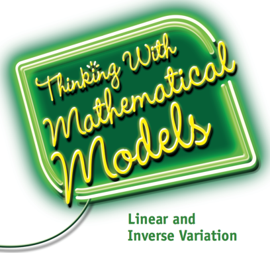8-1 Thinking with Mathematical Models

In Thinking With Mathematical Models, your child will model relationships with graphs and equations. They will use models to analyze situations and solve problems. The Investigations in this Unit will help them understand the following ideas.

• Represent data using graphs, tables, word descriptions and algebraic expressions.
• Recognize linear and nonlinear relationships in tables and graphs.
• Use linear and inverse variation equations to model bivariate data
• Use residual analysis to measure the fit of linear and inverse variation models.
• Analyze, approximate, and solve linear equations.
• Use linear and inverse variation equations to solve problems and to make predictions and decisions
• Use scatter plots, two-way tables, and correlation coefficients to describe patterns of association in pairs of variables.
• Use standard deviation to measure variability in data distributions.

When your child encounters a new problem, it is a good idea to ask questions such as:

• What are the key variables in this situation?
• If there is a pattern relating the variables, is it strong enough to allow me to make predictions?
• What is the pattern relating the variables?
• What kind of equation will express the relationship?
• How can I use the equation to answer questions about the relationship?

##8-2 Looking for Pythagoras

In Looking for Pythagoras, your child will explore an important relationship among the side lengths of a right triangle. They will learn how to:

• Develop strategies for finding the distance between two points on a coordinate grid
• Explain a proof of the Pythagorean Theorem
• Understand and use the Pythagorean Theorem to solve everyday problems
• Write fractions as repeating or terminating decimals
• Write decimals as fractions
• Recognize rational and irrational numbers
• Locate irrational numbers on a number line
• Relate the area of a square to its side length, and the volume of a cube to its side length
• Estimate square roots and cube roots

When your student encounters a new problem, it is a good idea to ask questions such as:

• What are the quantities in this problem?
• Is the Pythagorean Theorem useful and appropriate in this situation?
• How do I know?
• Do I need to find the distance between two points?
• How are the side length and the area of a square related?
• How can I estimate the square root or cube root of a number?

##8-3 Growing, Growing, Growing

In Growing, Growing, Growing, your child will explore Exponential Functions, one of the most important types of nonlinear relationships. The Investigations in this Unit will help them learn how to:

• Identify situations in which a quantity grows or decays exponentially
• Recognize the connections between the growth patterns in tables, graphs, and equations that represent exponential functions
• Construct equations to express the relationship between the variables in an exponential function in data tables, graphs, and problem situations
• Compare exponential and linear functions
• Develop and use rules for working with exponents, including scientific notation, to write and interpret equivalent expressions
• Solve problems about exponential growth and decay from a variety of different areas, including science and business

As your child works on the Problems in this Unit, ask questions about situations that involve nonlinear relationships such as:

• How can I recognize whether the relationship between the variables is an exponential function?
• What is the growth or decay factor?
• What equation models the data in the table, graph, or problem situation?
• What can I learn about this situation by studying a table or graph of the exponential function?
• How can I answer questions about the problem situation by studying a table, graph, or equation that represents the exponential function?

##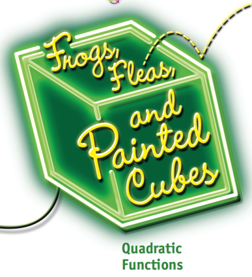8-4 Frogs, Fleas, and Painted Cubes

In Frogs, Fleas, and Painted Cubes, your child will explore quadratic functions, an important type of nonlinear function. They will learn how to

• Recognize patterns of change for quadratic relationships
• Write equations for quadratic functions represented in tables, graphs, and problem situations
• Connect quadratic equations to the patterns in tables and graphs of quadratic functions
• Use a quadratic equation to identify the maximum or minimum value, the x- and y-intercepts, line of symmetry, and other important features of the graph of a quadratic function
• Use the Distributive Property to write equivalent quadratic expressions in factored and expanded form
• Use tables, graphs, and equations of quadratic functions to solve problems in a variety of situations from geometry, science, and business
• Compare properties of quadratic, linear, and exponential functions

When your child encounters a new problem, it is a good idea to ask questions such as:

• What are the independent and dependent variables?
• How can I recognize whether the relationship between the variables is quadratic?
• What equation models a quadratic function given in a table, graph, or problem context?
• How can I answer questions about the problem situation by studying a table, graph, or equation representing a quadratic function?

##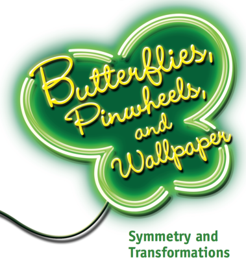8-5 Butterflies, Pinwheels and Wallpaper

In Butterflies, Pinwheels, and Wallpaper, your child will learn how to:

• Identify figures that have different kinds of symmetry
• Describe types of symmetry using reflections, rotations, and translations
• Use symmetry transformations to compare the size and shape of figures to see whether they are congruent or similar
• Identify congruent and similar triangles and quadrilaterals efficiently
• Use properties of congruent and similar triangles to solve problems about shapes and measurements

When your student encounters a new problem, it is a good idea to ask questions such as:

• How can I use symmetry to describe the shape and properties of figures in a design or a problem?
• What figures in a pattern are congruent?
• What parts of congruent figures will be matched by a congruence transformation?
• What figures in a problem are similar?

##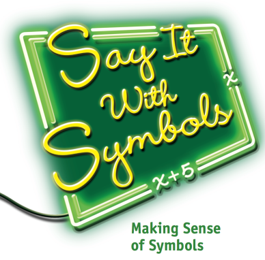8-6 Say It With Symbols

Algebra provides ideas and symbols for expressing information about quantitative variables and relationships. In Say It With Symbols, your child will solve problems designed to develop their understanding and skill in using symbolic expressions and equations in algebra. They will learn how to:

• Represent patterns and relationships in symbolic forms
• Determine when different symbolic expressions are mathematically equivalent
• Write algebraic expressions in useful equivalent forms
• Combine symbolic expressions using algebraic operations to form new expressions
• Analyze expressions or equations to determine the patterns of change in the tables and graphs that the expression or equation represents
• Solve linear and quadratic equations using symbolic reasoning
• Use algebraic reasoning to validate generalizations and conjectures

When your child encounters a new problem, it is a good idea to ask questions such as:

• What expression or equation represents the pattern or relationship in a context?
• Can you write an equivalent expression for a given expression to provide new information about a relationship?
• What operations can transform a given equation or expression into an equivalent form that can be used to answer a question?
• How can symbolic reasoning help confirm a conjecture?

##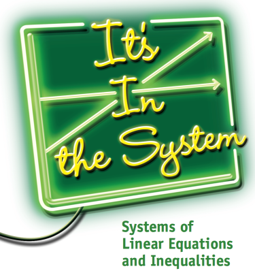8-7 It's in the System

In this Unit, your child will write and solve systems of linear equations and inequalities that model real-world situations. The methods for solving these algebraic systems combine graphic and algebraic reasoning from earlier Connected Mathematics Units. You will learn how to

• Solve linear equations and systems of linear equations with two variables
• Solve linear inequalities and systems of inequalities with two variables
• Use systems of linear equations and inequalities to solve problems

When your child encounters a new problem, it is a good idea to ask questions such as:

• What are the variables in this problem?
• Does the problem call for solving a system of equations or inequalities relating those variables?
• What strategy will be most effective in solving the system?

##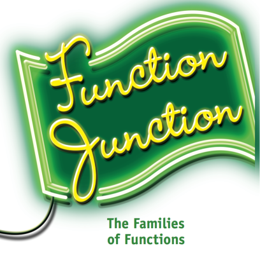8-8 Function Junction

Functions have been a major theme throughout your child’s work with algebra. In Function Junction your child will take a deeper look at functions and explore new functions. The Investigations in this Unit will help them learn how to:

• Determine the domain and range of functions and the f (x) notation for expressing functions
• Examine numeric and graphic properties of step and piecewise defined functions
• Investigate properties and uses of inverse functions
• Investigate properties and applications of arithmetic and geometric sequences
• Explore the relationships between functions with graphs connected by transformations such as translations and dilations
• Express quadratic functions in equivalent vertex form and use that new form to solve equations and sketch graphs
• Develop a formula for solving any quadratic equation
• Explore the meaning and operations of complex numbers
• Use polynomial expressions and functions to model and to answer questions about data patterns and graphs that cannot be represented with linear, quadratic, inverse variation, or exponential functions

As they work through the Problems of this Unit, it will be helpful to keep asking questions such as:

• What are the variables in the situation and how are they related?
• What familiar type of function could be used to model the relationship of variables, or is something new required?
• How are algebraic expressions and graphs of the relationship between variables connected to each other?
• How can the algebraic expression for a function be written in a form that makes it easier to sketch or analyze a graph or solve an equation?